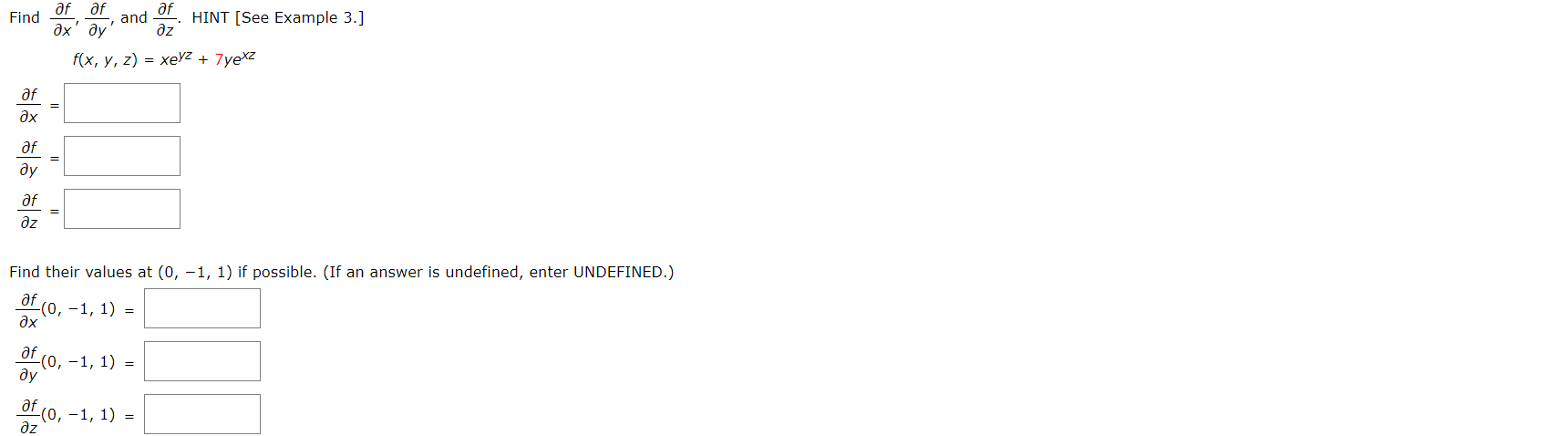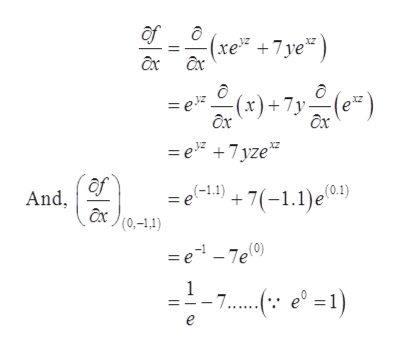# af afafHINT [See Example 3.]Findandдх дуdzf(x, y, z) = xeyZ + 7yexzafдхafдудfдzFind their values at (0, -1, 1) if possible. (If an answer is undefined, enter UNDEFINED.)дf(0, -1, 1) =дхaf(0,-1, 1) =ду-(0, -1, 1) =дz

Question
2 viewshelp_outlineImage Transcriptioncloseaf af af HINT [See Example 3.] Find and дх ду dz f(x, y, z) = xeyZ + 7yexz af дх af ду дf дz Find their values at (0, -1, 1) if possible. (If an answer is undefined, enter UNDEFINED.) дf (0, -1, 1) = дх af (0,-1, 1) = ду -(0, -1, 1) = дz fullscreen
check_circle

Step 1

The given function is

Step 2

To find: The value of partial derivatives of function with rerspect to x, y and z. And then evaluate partial derivative at point (0,-1,1).

Step 3
• The partial differentiation of (1) wi...help_outlineImage Transcriptioncloseof (xe +7ye* ) (*) + 7y (e*) = ez ôx = e" +7 yze* (ro) = e-1.1) + 7(-1.1)e And, (0,-1,1) = e - 7e(0) - 7..: e° =1) - 7....(: e° =1) fullscreen

### Want to see the full answer?

See Solution

#### Want to see this answer and more?

Solutions are written by subject experts who are available 24/7. Questions are typically answered within 1 hour.*

See Solution
*Response times may vary by subject and question.
Tagged in

### Derivative